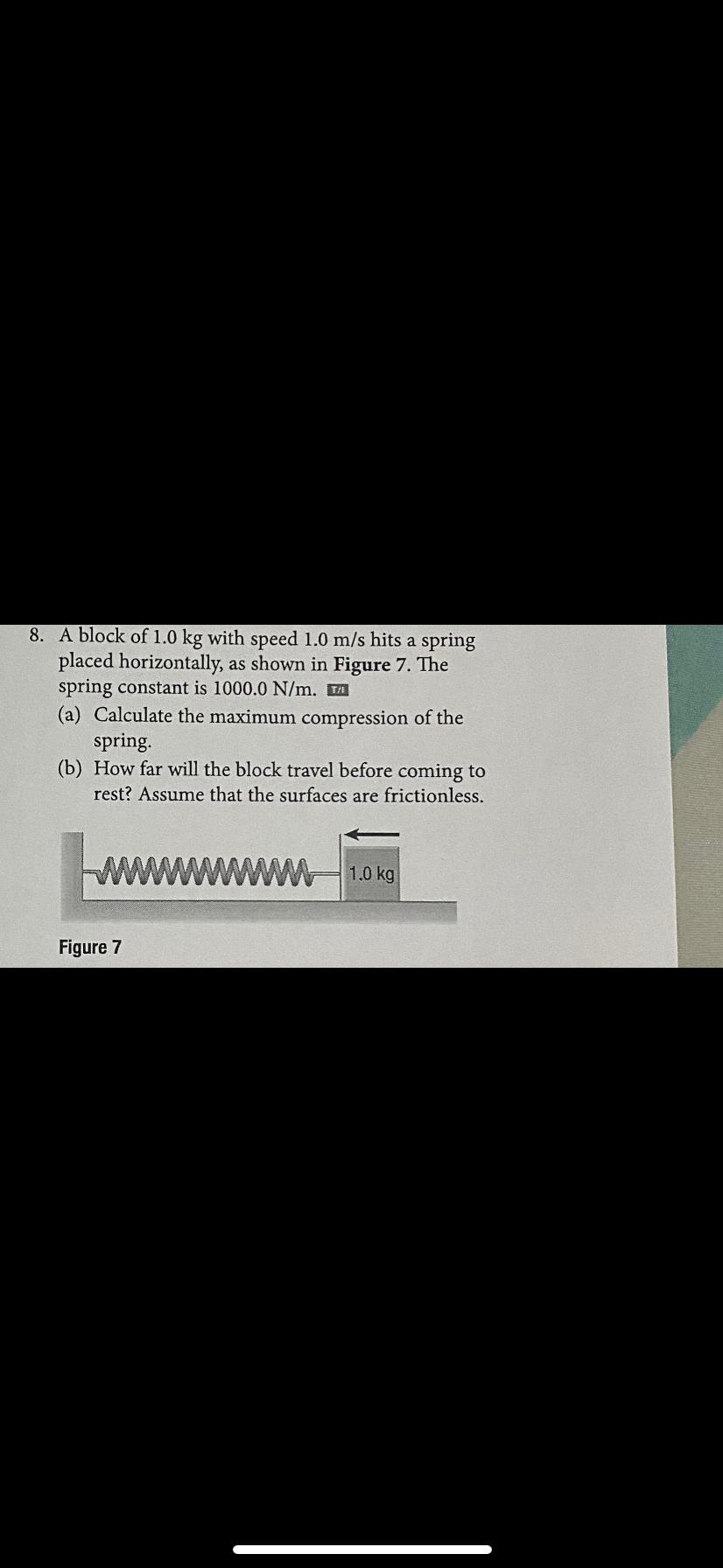Question:

# A block of 1.0 kg with speed 1.0 m/s hits a spring placed horizontally, as shown in Figure 7. The spring constant is 1000.0 N/m. (a) Calculate the maximum compression of the spring. (b) How far willA block of 1.0 kg with speed 1.0 m/s hits a spring placed horizontally, as shown in Figure 7. The spring constant is 1000.0 N/m. (a) Calculate the maximum compression of the spring. (b) How far will the block travel before coming to rest? Assume that the surfaces are frictionless.